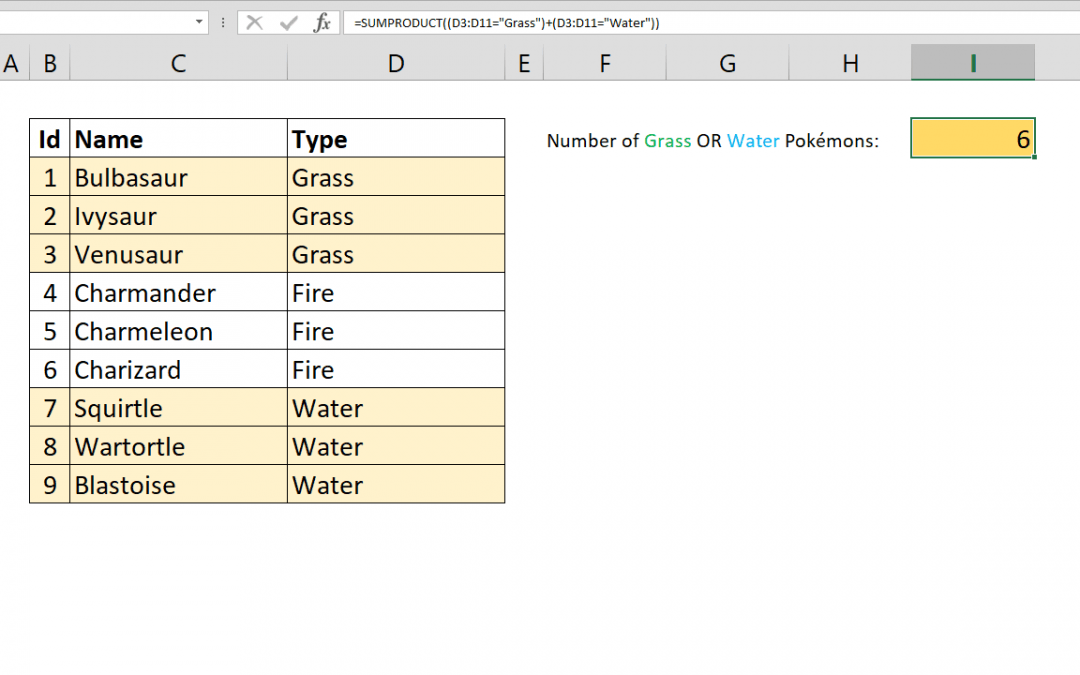## How to calculate average of values with OR operator

The AVERAGEIFS function can calculate the average of values with multiple conditions. However, these conditions are linked with AND operator. But if you want to handle AVERAGE OR combination, AVERAGEIFS function will not work. This article shows how to calculate...## How to count values with OR operator

You can count values in Excel by multiple criteria using the COUNTIFS function. However COUNTIFS will only link those criterion by AND operation. If you want to COUNTIFS OR, you need an alternative approach. This article shows how to count values with OR operator...## How to count characters in Excel in a range

The LEN function is used to count characters in Excel. But it doesn’t support ranges or arrays to easily count total number of characters in a range. This article shows 2 ways to count characters in Excel in a range which uses SUMPRODUCT and SUM along with LEN....## How to calculate Basic Tax Rate with VLOOKUP

The tax brackets can be confusing to work with. Different ‘levels’ of your income needs to be multiplied with a different percentage to find the total. This can be done with the conventional Excel formulas like IF and a bunch of others, but that’s the long and hard to...## How to check if a value exists in a list

The logical tests and conditional checks have important role in Excel models. Potential errors can be detected and handled by using the right logical tests. If you know How to check if a value exists in a list, you can use this logical statement to detect and...## How to count values by length

This article shows how to count values by length based on their character count. This method can be helpful to check your data against invalid entries. It is also a good practice to use SUMPRODUCT and N functions together. Syntax =SUMPRODUCT(N(LEN(range)>character...